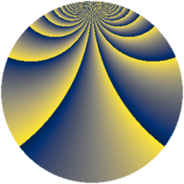# Properties

 Label 2240.2.dxLevel $2240$ Weight $2$ Character orbit 2240.dx Rep. character $\chi_{2240}(141,\cdot)$ Character field $\Q(\zeta_{16})$ Dimension $1536$ Sturm bound $768$

# Related objects

## Defining parameters

 Level: $$N$$ $$=$$ $$2240 = 2^{6} \cdot 5 \cdot 7$$ Weight: $$k$$ $$=$$ $$2$$ Character orbit: $$[\chi]$$ $$=$$ 2240.dx (of order $$16$$ and degree $$8$$) Character conductor: $$\operatorname{cond}(\chi)$$ $$=$$ $$64$$ Character field: $$\Q(\zeta_{16})$$ Sturm bound: $$768$$

## Dimensions

The following table gives the dimensions of various subspaces of $$M_{2}(2240, [\chi])$$.

Total New Old
Modular forms 3104 1536 1568
Cusp forms 3040 1536 1504
Eisenstein series 64 0 64

## Trace form

 $$1536q + O(q^{10})$$ $$1536q + 16q^{22} + 160q^{32} + 160q^{34} + 16q^{44} + 64q^{51} - 128q^{54} - 16q^{56} - 288q^{58} - 192q^{62} + 160q^{63} + 160q^{67} - 192q^{68} - 288q^{72} - 16q^{74} - 128q^{76} + 96q^{78} + 64q^{79} + 128q^{80} + 416q^{86} + 320q^{88} + 32q^{94} + O(q^{100})$$

## Decomposition of $$S_{2}^{\mathrm{new}}(2240, [\chi])$$ into newform subspaces

The newforms in this space have not yet been added to the LMFDB.

## Decomposition of $$S_{2}^{\mathrm{old}}(2240, [\chi])$$ into lower level spaces

$$S_{2}^{\mathrm{old}}(2240, [\chi]) \cong$$ $$S_{2}^{\mathrm{new}}(64, [\chi])$$$$^{\oplus 4}$$$$\oplus$$$$S_{2}^{\mathrm{new}}(320, [\chi])$$$$^{\oplus 2}$$$$\oplus$$$$S_{2}^{\mathrm{new}}(448, [\chi])$$$$^{\oplus 2}$$